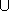## CGAL::Topological_explorer

### Definition

An instance D of the data type Topological_explorer is a decorator for interfacing the topological structure of a plane map P (read-only).

A plane map P consists of a triple (V, E, F) of vertices, edges, and faces. We collectively call them objects. An edge e is a pair of vertices (v,w) with incidence operations v = source(e), w = target(e). The list of all edges with source v is called the adjacency list A(v).

Edges are paired into twins. For each edge e = (v,w) there's an edge twin(e) = (w,v) and twin(twin(e)) == e1.

An edge e = (v,w) knows two adjacent edges en = next(e) and ep = previous(e) where source(en) = w, previous(en) = e and target(ep) = v and next(ep) = e. By this symmetric previous-next relationship all edges are partitioned into face cycles. Two edges e and e' are in the same face cycle if e = next*(e'). All edges e in the same face cycle have the same incident face f = face(e). The cyclic order on the adjacency list of a vertex v = source(e) is given by cyclic_adj_succ(e) = twin(previous(e)) and cyclic_adj_pred(e) = next(twin(e)).

A vertex v is embedded via coordinates point(v). By the embedding of its source and target an edge corresponds to a segment. P has the property that the embedding is always order-preserving. This means a ray fixed in point(v) of a vertex v and swept around counterclockwise meets the embeddings of target(e) (eA(v)) in the cyclic order defined by the list order of A.

The embedded face cycles partition the plane into maximal connected subsets of points. Each such set corresponds to a face. A face is bounded by its incident face cycles. For all the edges in the non-trivial face cycles it holds that the face is left of the edges. There can also be trivial face cycles in form of isolated vertices in the interior of a face. Each such vertex v knows its surrounding face f = face(v).

Plane maps are attributed, for each object uVEF we attribute an information mark(u) of type Mark. Mark fits the concepts assignable, default-constructible, and equal-comparable.

### Types

 Topological_explorer::Plane_map The underlying plane map type Topological_explorer::Point The point type of vertices. Topological_explorer::Mark All objects (vertices, edges, faces) are attributed by a Mark object. Topological_explorer::Size_type The size type.

Local types are handles, iterators and circulators of the following kind: Vertex_const_handle, Vertex_const_iterator, Halfedge_const_handle, Halfedge_const_iterator, Face_const_handle, Face_const_iterator. Additionally the following circulators are defined.

 Topological_explorer::Halfedge_around_vertex_const_circulator circulating the outgoing halfedges in A(v). Topological_explorer::Halfedge_around_face_const_circulator circulating the halfedges in the face cycle of a face f. Topological_explorer::Hole_const_iterator iterating all holes of a face f. The type is convertible to Halfedge_const_handle. Topological_explorer::Isolated_vertex_const_iterator iterating all isolated vertices of a face f. The type generalizes Vertex_const_handle.

### Operations

 Vertex_const_handle D.source ( Halfedge_const_handle e) returns the source of e. Vertex_const_handle D.target ( Halfedge_const_handle e) returns the target of e. Halfedge_const_handle D.twin ( Halfedge_const_handle e) returns the twin of e. bool D.is_isolated ( Vertex_const_handle v) returns true iff A(v) =. Halfedge_const_handle D.first_out_edge ( Vertex_const_handle v) returns one halfedge with source v. It's the starting point for the circular iteration over the halfedges with source v. Precondition: !is_isolated(v). Halfedge_const_handle D.last_out_edge ( Vertex_const_handle v) returns the halfedge with source v that is the last in the circular iteration before encountering first_out_edge(v) again. Precondition: !is_isolated(v). Halfedge_const_handle D.cyclic_adj_succ ( Halfedge_const_handle e) returns the edge after e in the cyclic ordered adjacency list of source(e). Halfedge_const_handle D.cyclic_adj_pred ( Halfedge_const_handle e) returns the edge before e in the cyclic ordered adjacency list of source(e). Halfedge_const_handle D.next ( Halfedge_const_handle e) returns the next edge in the face cycle containing e. Halfedge_const_handle D.previous ( Halfedge_const_handle e) returns the previous edge in the face cycle containing e. Face_const_handle D.face ( Halfedge_const_handle e) returns the face incident to e. Face_const_handle D.face ( Vertex_const_handle v) returns the face incident to v. Precondition: is_isolated(v). Halfedge_const_handle D.halfedge ( Face_const_handle f) returns a halfedge in the bounding face cycle of f (Halfedge_const_handle() if there is no bounding face cycle).

### Iteration

 Vertex_const_iterator D.vertices_begin () iterator over vertices of the map. Vertex_const_iterator D.vertices_end () past-the-end iterator for vertices. Halfedge_const_iterator D.halfedges_begin () iterator over halfedges of the map. Halfedge_const_iterator D.halfedges_end () past-the-end iterator for halfedges. Face_const_iterator D.faces_begin () iterator over faces of the map. Face_const_iterator D.faces_end () past-the-end iterator for faces Halfedge_around_vertex_const_circulator D.out_edges ( Vertex_const_handle v) returns a circulator for the cyclic adjacency list of v. Halfedge_around_face_const_circulator D.face_cycle ( Face_const_handle f) returns a circulator for the outer face cycle of f. Hole_const_iterator D.holes_begin ( Face_const_handle f) returns an iterator for all holes in the interior of f. A Hole_iterator can be assigned to a Halfedge_around_face_const_circulator. Hole_const_iterator D.holes_end ( Face_const_handle f) returns the past-the-end iterator of f. Isolated_vertex_const_iterator D.isolated_vertices_begin ( Face_const_handle f) returns an iterator for all isolated vertices in the interior of f. Isolated_vertex_const_iterator D.isolated_vertices_end ( Face_const_handle f) returns the past the end iterator of f.

### Associated Information

The type Mark is the general attribute of an object.

 Point D.point ( Vertex_const_handle v) returns the embedding of v. Mark D.mark ( Vertex_const_handle v) returns the mark of v. Mark D.mark ( Halfedge_const_handle e) returns the mark of e. Mark D.mark ( Face_const_handle f) returns the mark of f.

### Statistics and Integrity

Size_type D.number_of_vertices ()
returns the number of vertices.

Size_type D.number_of_halfedges ()
returns the number of halfedges.

Size_type D.number_of_edges ()
returns the number of halfedge pairs.

Size_type D.number_of_faces ()
returns the number of faces.

Size_type D.number_of_face_cycles ()
returns the number of face cycles.

Size_type D.number_of_connected_components ()
calculates the number of connected components of P.

void D.print_statistics ( std::ostream& os = std::cout)
print the statistics of P: the number of vertices, edges, and faces.

void
 D.check_integrity_and_topological_planarity ( bool faces=true)
checks the link structure and the genus of P.

### Footnotes

 1 The existence of the edge pairs makes P a bidirected graph, the twin links make P a map.